Resources tagged with: GeoGebra

Filter by: Content type:
Age range:
Challenge level:

There are 90 results

Broad Topics > Physical and Digital Manipulatives > GeoGebraWhere Is the Dot?

Age 14 to 16Challenge Level

A dot starts at the point (1,0) and turns anticlockwise. Can you estimate the height of the dot after it has turned through 45 degrees? Can you calculate its height?Triangle in a Triangle

Age 14 to 16Challenge Level

Can you work out the fraction of the original triangle that is covered by the inner triangle?Round and Round a Circle

Age 14 to 16Challenge Level

Can you explain what is happening and account for the values being displayed?Pythagoras Proofs

Age 14 to 16Challenge Level

Can you make sense of these three proofs of Pythagoras' Theorem?Points in Pairs

Age 14 to 16Challenge Level

Move the point P to see how P' moves. Then use your insights to calculate a missing length.Sine and Cosine

Age 14 to 16Challenge Level

The sine of an angle is equal to the cosine of its complement. Can you explain why and does this rule extend beyond angles of 90 degrees?Sine and Cosine for Connected Angles

Age 14 to 16Challenge Level

The length AM can be calculated using trigonometry in two different ways. Create this pair of equivalent calculations for different peg boards, notice a general result, and account for it.Shear Magic

Age 11 to 14Challenge Level

Explore the area of families of parallelograms and triangles. Can you find rules to work out the areas?Using Geogebra

Age 11 to 18Mixing Paints

Age 11 to 14Challenge Level

Can you work out how to produce different shades of pink paint?The Medieval Octagon

Age 14 to 16Challenge Level

Medieval stonemasons used a method to construct octagons using ruler and compasses... Is the octagon regular? Proof please.Age 14 to 16Challenge LevelSame Length

Age 11 to 16Challenge Level

Construct two equilateral triangles on a straight line. There are two lengths that look the same - can you prove it?Squirty

Age 14 to 16Challenge Level

Using a ruler, pencil and compasses only, it is possible to construct a square inside any triangle so that all four vertices touch the sides of the triangle.Overlap

Age 11 to 16Challenge Level

A red square and a blue square overlap. Does the red square always cover a quarter of the blue square?Tessellation Interactivity

Age 7 to 16Challenge Level

An environment that enables you to investigate tessellations of regular polygonsMixing More Paints

Age 14 to 16Challenge Level

Can you find an efficent way to mix paints in any ratio?Rolling Around

Age 11 to 14Challenge Level

A circle rolls around the outside edge of a square so that its circumference always touches the edge of the square. Can you describe the locus of the centre of the circle?Squaring the Circle and Circling the Square

Age 14 to 16Challenge Level

If you continue the pattern, can you predict what each of the following areas will be? Try to explain your prediction.Age 14 to 16Challenge Level

The points P, Q, R and S are the midpoints of the edges of a non-convex quadrilateral.What do you notice about the quadrilateral PQRS and its area?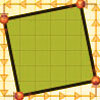At Right Angles

Age 14 to 16Challenge Level

Can you decide whether two lines are perpendicular or not? Can you do this without drawing them?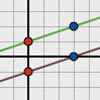Parallel Lines

Age 11 to 14Challenge Level

How does the position of the line affect the equation of the line? What can you say about the equations of parallel lines?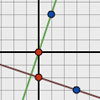Perpendicular Lines

Age 14 to 16Challenge Level

Position the lines so that they are perpendicular to each other. What can you say about the equations of perpendicular lines?The Farmers' Field Boundary

Age 11 to 14Challenge Level

The farmers want to redraw their field boundary but keep the area the same. Can you advise them?Isosceles Triangles

Age 11 to 14Challenge Level

Draw some isosceles triangles with an area of $9$cm$^2$ and a vertex at (20,20). If all the vertices must have whole number coordinates, how many is it possible to draw?Bow Tie

Age 11 to 14Challenge Level

Show how this pentagonal tile can be used to tile the plane and describe the transformations which map this pentagon to its images in the tiling.A Tilted Square

Age 14 to 16Challenge Level

The opposite vertices of a square have coordinates (a,b) and (c,d). What are the coordinates of the other vertices?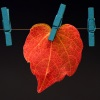Number Lines in Disguise

Age 7 to 14Challenge Level

Some of the numbers have fallen off Becky's number line. Can you figure out what they were?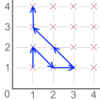Areas from Vectors

Age 11 to 16

Use the applet to explore the area of a parallelogram and how it relates to vectors.Coordinates of Corners

Age 11 to 16

Use the applet to make some squares. What patterns do you notice in the coordinates?Farey Sequences

Age 11 to 14Challenge Level

There are lots of ideas to explore in these sequences of ordered fractions.Tilting Triangles

Age 14 to 16Challenge Level

A right-angled isosceles triangle is rotated about the centre point of a square. What can you say about the area of the part of the square covered by the triangle as it rotates?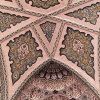Angles Inside

Age 11 to 14Challenge Level

Draw some angles inside a rectangle. What do you notice? Can you prove it?Age 14 to 16Challenge Level

Make five different quadrilaterals on a nine-point pegboard, without using the centre peg. Work out the angles in each quadrilateral you make. Now, what other relationships you can see?Spinners Environment

Age 5 to 18Challenge Level

A tool for generating random integers.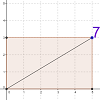Exploring Diagonals

Age 11 to 16

Move the corner of the rectangle. Can you work out what the purple number represents?Beelines

Age 14 to 16Challenge Level

Is there a relationship between the coordinates of the endpoints of a line and the number of grid squares it crosses?More Twisting and Turning

Age 11 to 16Challenge Level

It would be nice to have a strategy for disentangling any tangled ropes...Magic Potting Sheds

Age 11 to 16Challenge Level

Mr McGregor has a magic potting shed. Overnight, the number of plants in it doubles. He'd like to put the same number of plants in each of three gardens, planting one garden each day. Can he do it?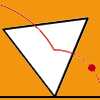Speeding Up, Slowing Down

Age 11 to 14Challenge Level

Experiment with the interactivity of "rolling" regular polygons, and explore how the different positions of the red dot affects its speed at each stage.Solving Together - Estimating Angles

Age 11 to 14

Week 2
How well can you estimate angles? Playing this game could improve your skills.Right Angles

Age 11 to 14Challenge Level

Can you make a right-angled triangle on this peg-board by joining up three points round the edge?Subtended Angles

Age 11 to 14Challenge Level

What is the relationship between the angle at the centre and the angles at the circumference, for angles which stand on the same arc? Can you prove it?Square Coordinates

Age 11 to 14Challenge Level

A tilted square is a square with no horizontal sides. Can you devise a general instruction for the construction of a square when you are given just one of its sides?Factor Lines

Age 7 to 14Challenge Level

Arrange the four number cards on the grid, according to the rules, to make a diagonal, vertical or horizontal line.Reflecting Squarely

Age 11 to 14Challenge Level

In how many ways can you fit all three pieces together to make shapes with line symmetry?Guesswork

Age 14 to 16Challenge Level

Ask a friend to choose a number between 1 and 63. By identifying which of the six cards contains the number they are thinking of it is easy to tell them what the number is.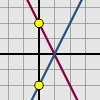Reflecting Lines

Age 11 to 14Challenge Level

Investigate what happens to the equations of different lines when you reflect them in one of the axes. Try to predict what will happen. Explain your findings.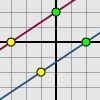Translating Lines

Age 11 to 14Challenge Level

Investigate what happens to the equation of different lines when you translate them. Try to predict what will happen. Explain your findings.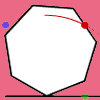Up and Across

Age 11 to 14Challenge Level

Experiment with the interactivity of "rolling" regular polygons, and explore how the different positions of the dot affects its vertical and horizontal movement at each stage.